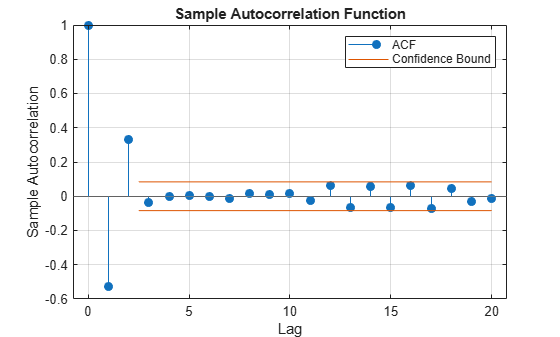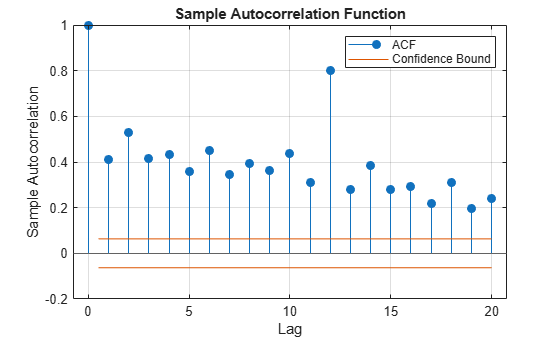autocorr

Sample autocorrelation

Description

example

autocorr(y) plots the sample autocorrelation function (ACF) of the univariate, stochastic time series y with confidence bounds.

example

autocorr(y,Name,Value) uses additional options specified by one or more name-value pair arguments. For example, autocorr(y,'NumLags',10,'NumSTD',2) plots the sample ACF of y for 10 lags and displays confidence bounds consisting of 2 standard errors.

example

acf = autocorr(___) returns the sample ACF of y using any of the input arguments in the previous syntaxes.

example

[acf,lags,bounds] = autocorr(___) additionally returns the lag numbers that MATLAB® uses to compute the ACF, and also returns the approximate upper and lower confidence bounds.

autocorr(ax,___) plots on the axes specified by ax instead of the current axes (gca). ax can precede any of the input argument combinations in the previous syntaxes.

[acf,lags,bounds,h] = autocorr(___) plots the sample ACF of y and additionally returns handles to plotted graphics objects. Use elements of h to modify properties of the plot after you create it.

Examples

collapse all

Specify the MA(2) model:

${y}_{t}={\epsilon }_{t}-0.5{\epsilon }_{t-1}+0.4{\epsilon }_{t-2},$

where ${\epsilon }_{t}$ is Gaussian with mean 0 and variance 1.

rng(1); % For reproducibility
Mdl = arima('MA',{-0.5 0.4},'Constant',0,'Variance',1)
Mdl =
arima with properties:

Description: "ARIMA(0,0,2) Model (Gaussian Distribution)"
Distribution: Name = "Gaussian"
P: 0
D: 0
Q: 2
Constant: 0
AR: {}
SAR: {}
MA: {-0.5 0.4} at lags [1 2]
SMA: {}
Seasonality: 0
Beta: [1×0]
Variance: 1

Simulate 1000 observations from Mdl.

y = simulate(Mdl,1000);

Compute the ACF for 20 lags. Specify that ${\mathit{y}}_{\mathit{t}}$ is an MA(2) model, that is, the ACF is effectively 0 after the second lag.

[acf,lags,bounds] = autocorr(y,'NumMA',2);
bounds
bounds = 2×1

0.0843
-0.0843

bounds is (-0.0843, 0.0843), which are the upper and lower confidence bounds.

Plot the ACF.

autocorr(y)The ACF cuts off after the second lag. This behavior is indicative of an MA(2) process.

Specify the multiplicative seasonal ARMA $\left(2,0,1\right)×\left(3,0,0{\right)}_{12}$ model:

$\left(1-0.75L-0.15{L}^{2}\right)\left(1-0.9{L}^{12}+0.5{L}^{24}-0.5{L}^{36}\right){y}_{t}=2+{\epsilon }_{t}-0.5{\epsilon }_{t-1},$

where ${\epsilon }_{t}$ is Gaussian with mean 0 and variance 1.

Mdl = arima('AR',{0.75,0.15},'SAR',{0.9,-0.5,0.5},...
'SARLags',[12,24,36],'MA',-0.5,'Constant',2,...
'Variance',1);

Simulate data from Mdl.

rng(1); % For reproducibility
y = simulate(Mdl,1000);

Plot the default autocorrelation function (ACF).

figure
autocorr(y)The default correlogram does not display the dependence structure for higher lags.

Plot the ACF for 40 lags.

figure
autocorr(y,'NumLags',40,'NumSTD',3)The correlogram shows the larger correlations at lags 12, 24, and 36.

Although various estimates of the sample autocorrelation function exist, autocorr uses the form in Box, Jenkins, and Reinsel, 1994. In their estimate, they scale the correlation at each lag by the sample variance (var(y,1)) so that the autocorrelation at lag 0 is unity. However, certain applications require rescaling the normalized ACF by another factor.

Simulate 1000 observations from the standard Gaussian distribution.

rng(1); % For reproducibility
y = randn(1000, 1);

Compute the normalized and unnormalized sample ACF.

[normalizedACF, lags] = autocorr(y,'NumLags',10);
unnormalizedACF = normalizedACF*var(y,1);

Compare the first 10 lags of the sample ACF with and without normalization.

[lags  normalizedACF  unnormalizedACF]
ans = 11×3

0    1.0000    0.9960
1.0000   -0.0180   -0.0180
2.0000    0.0536    0.0534
3.0000   -0.0206   -0.0205
4.0000   -0.0300   -0.0299
5.0000   -0.0086   -0.0086
6.0000   -0.0108   -0.0107
7.0000   -0.0116   -0.0116
8.0000    0.0309    0.0307
9.0000    0.0341    0.0340
⋮

Input Arguments

collapse all

Observed univariate time series for which MATLAB estimates or plots the ACF, specified as a numeric vector. The last element of y contains the latest observation.

Specify missing observations using NaN. The autocorr function treats missing values as missing completely at random.

Data Types: double

Axes on which to plot, specified as an Axes object.

By default, autocorr plots to the current axes (gca).

Name-Value Arguments

Specify optional comma-separated pairs of Name,Value arguments. Name is the argument name and Value is the corresponding value. Name must appear inside quotes. You can specify several name and value pair arguments in any order as Name1,Value1,...,NameN,ValueN.

Example: autocorr(y,'NumLags',10,'NumSTD',2) plots the sample ACF of y for 10 lags and displays confidence bounds consisting of 2 standard errors.

Number of lags in the sample ACF, specified as the comma-separated pair consisting of 'NumLags' and a positive integer. autocorr uses lags 0:NumLags to estimate the ACF.

The default is min([20,T – 1]), where T is the effective sample size of y.

Example: autocorr(y,'NumLags',10) plots the sample ACF of y for lags 0 through 10.

Data Types: double

Number of lags in a theoretical MA model of y, specified as the comma-separated pair consisting of 'NumMA' and a nonnegative integer less than NumLags.

autocorr uses NumMA to estimate confidence bounds.

• For lags > NumMA, autocorr uses Bartlett’s approximation  to estimate the standard errors under the model assumption.

• If NumMA = 0, then autocorr assumes that y is a Gaussian white-noise process of length n. Consequently, the standard error is approximately $1/\sqrt{T},$ where T is the effective sample size of y.

Example: autocorr(y,'NumMA',10) specifies that y is an MA(10) process, and plots confidence bounds for all lags greater than 10.

Data Types: double

Number of standard errors in the confidence bounds, specified as the comma-separated pair consisting of 'NumSTD' and a nonnegative scalar. For all lags > NumMA, the confidence bounds are 0 ±NumSTD*$\stackrel{^}{\sigma }$, where $\stackrel{^}{\sigma }$ is the estimated standard error of the sample autocorrelation.

The default yields approximate 95% confidence bounds.

Example: autocorr(y,'NumSTD',1.5) plots the ACF of y with confidence bounds 1.5 standard errors away from 0.

Data Types: double

Output Arguments

collapse all

Sample ACF of the univariate time series y, returned as a numeric vector of length NumLags + 1.

The elements of acf correspond to lags 0,1,2,...,NumLags (that is, elements of lags). For all time series y, the lag 0 autocorrelation acf(1) = 1.

Lag numbers used for ACF estimation, returned as a numeric vector of length NumLags + 1.

Approximate upper and lower autocorrelation confidence bounds assuming y is an MA(NumMA) process, returned as a two-element numeric vector.

Handles to plotted graphics objects, returned as a graphics array. h contains unique plot identifiers, which you can use to query or modify properties of the plot.

collapse all

Autocorrelation Function

The autocorrelation function measures the correlation between yt and yt + k, where k = 0,...,K and yt is a stochastic process.

According to , the autocorrelation for lag k is

${r}_{k}=\frac{{c}_{k}}{{c}_{0}},$

where

• ${c}_{k}=\frac{1}{T}\sum _{t=1}^{T-k}\left({y}_{t}-\overline{y}\right)\left({y}_{t+k}-\overline{y}\right).$

• c0 is the sample variance of the time series.

Suppose that q is the lag beyond which the theoretical ACF is effectively 0. Then, the estimated standard error of the autocorrelation at lag k > q is

$SE\left({r}_{k}\right)=\sqrt{\frac{1}{T}\left(1+2\sum _{j=1}^{q}{r}_{j}^{2}\right)}.$

If the series is completely random, then the standard error reduces to $1/\sqrt{T}$.

Missing Completely at Random

Observations of a random variable are missing completely at random if the tendency of an observation to be missing is independent of both the random variable and the tendency of all other observations to be missing.

Tips

To plot the ACF without confidence bounds, set 'NumSTD',0.

Algorithms

• If y is a fully observed series (that is, it does not contain any NaN values), then autocorr uses a Fourier transform to compute the ACF in the frequency domain, then converts back to the time domain using an inverse Fourier transform.

• If y is not fully observed (that is, it contains at least one NaN value), autocorr computes the ACF at lag k in the time domain, and includes in the sample average only those terms for which the cross product ytyt+k exists. Consequently, the effective sample size is a random variable.

• autocorr plots the ACF when you do not request any output or when you request the fourth output.

 Box, G. E. P., G. M. Jenkins, and G. C. Reinsel. Time Series Analysis: Forecasting and Control. 3rd ed. Englewood Cliffs, NJ: Prentice Hall, 1994.

 Hamilton, J. D. Time Series Analysis. Princeton, NJ: Princeton University Press, 1994.